• +91 9971497814
• info@interviewmaterial.com

# RD Chapter 6- Trigonometric Identities Ex-6.1 Interview Questions Answers

### Related Subjects

Question 1 : Prove the following trigonometric identities :
(1 – cos2 A) cosec2 A = 1

(1 – cos2 A)cosec2 A = 1
L.H.S. = (1 – cos2 A) cosec2 A = sin2 Acosec2 A  (
1 – cos2 A = sin2 A)
= (sin A cosec A)2 = (l)2 = 1 = R.H.S. {sin A cosec A = 1 }

Question 2 : (1 + cot2 A) sin2 A = 1

(1 + cot2 A)sin2 A = 1
L.H.S. = (1 + cot2 A) (sin2 A)
= cosec2 A sin2 A {1 + cot2 A =cosec2 A}
= [cosec A sin A]2
= (1)2= 1 = R.H.S. (
sin A cosec A = 1

Question 3 : tan2 θ cos2  θ = 1- cos2  θ

Answer 3 :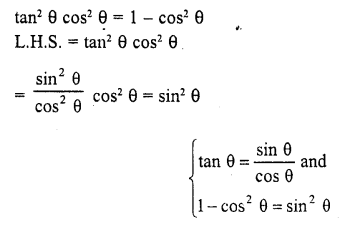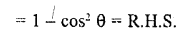Question 4 :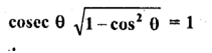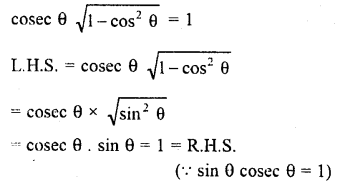Question 5 : (sec2 θ – 1) (cosec2 θ – 1) = 1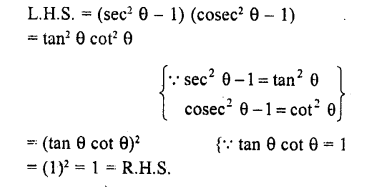Question 6 :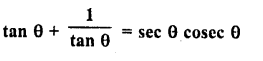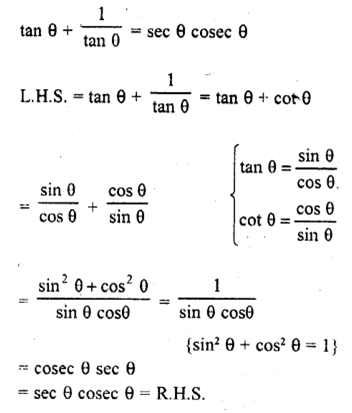Question 7 :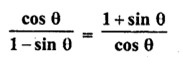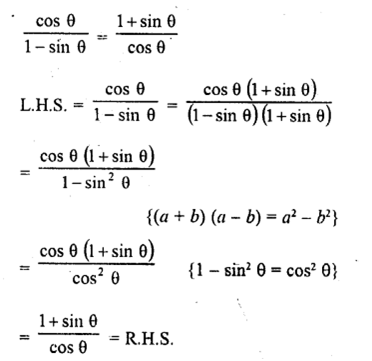Question 8 :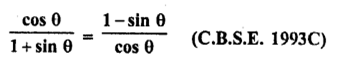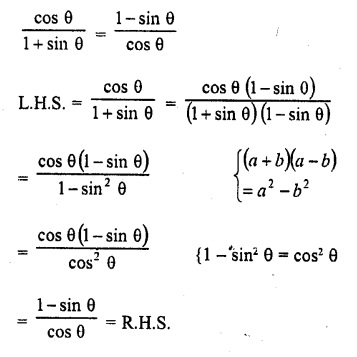Question 9 :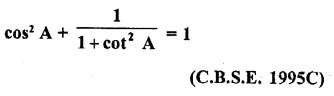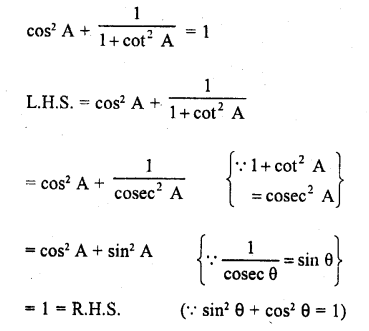Question 10 :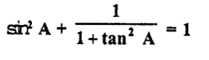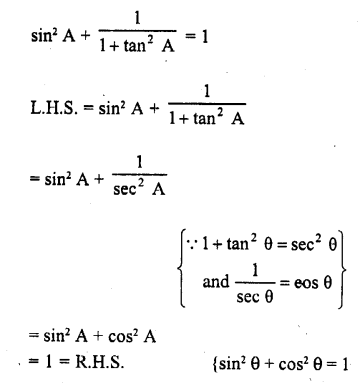Todays Deals### RD Chapter 6- Trigonometric Identities Ex-6.1 Contributorskrishan

Name:
Email:

# Latest News# 9000 interview questions in different categories